# From Gen Chem to Organic Chem, Pt. 13 – Equilibria

Last updated: February 20th, 2020 |

(The last lesson in the series. I wish I had this series done by the time school started for most people, but I initially thought I could cover the basics of Gen Chem in 6 posts and it just kept growing – planning fallacy in action.)

Chemical Equilibria

In chemistry, irreversible reactions get all the press:  the familiar fires and explosions that are well represented on YouTube.  The thing is, not all reactions go to completion. Sometimes, the products of a reaction can react together and regenerate the starting materials, thereby setting up a reversible reaction. These are the types of reaction that are in action all around us and are so nondescript we never take notice. In any given moment, a mind-boggling number of protons in my Diet Coke (pH 3.39) are exchanging with water molecules, but since there is no net change, the process is practically invisible.

For the last subject in “From Gen Chem to Org Chem”, we’re going to talk about chemical equilibrium, which plays a huge topic in organic chemistry.  A lot of the reactions you will learn about are equilibrium reactions, and this concept extends beyond chemical reactions to a whole number of processes, like how substrates bind to enzymes and even how molecules reversibly change their three-dimensional structure.

So what’s chemical equilibrium anyway? Why is it important? What does it mean to say that we have chemical equilibrium?

Let’s start off with the basics:

• A chemical equilibrium can only occur in a situation where there is a significant reverse reaction.
• When chemical equilibrium is established, there is no change in the concentrations of the reactants and products over time.
• At equilibrium, the delta G of the process is equal to zero. There is no driving force.

Probably the most important way you’re introduced to equilibria in Gen Chem is through acid-base reactions. The reaction of the strong acid hydrochloric acid (HCl) with water produces H3O(+), which is essentially an irreversible reaction. In contrast, the weak acid acetic acid (CH3COOH) reacts with H2O to give H3O(+) and CH3COO(–), but the reverse reaction can happen as well.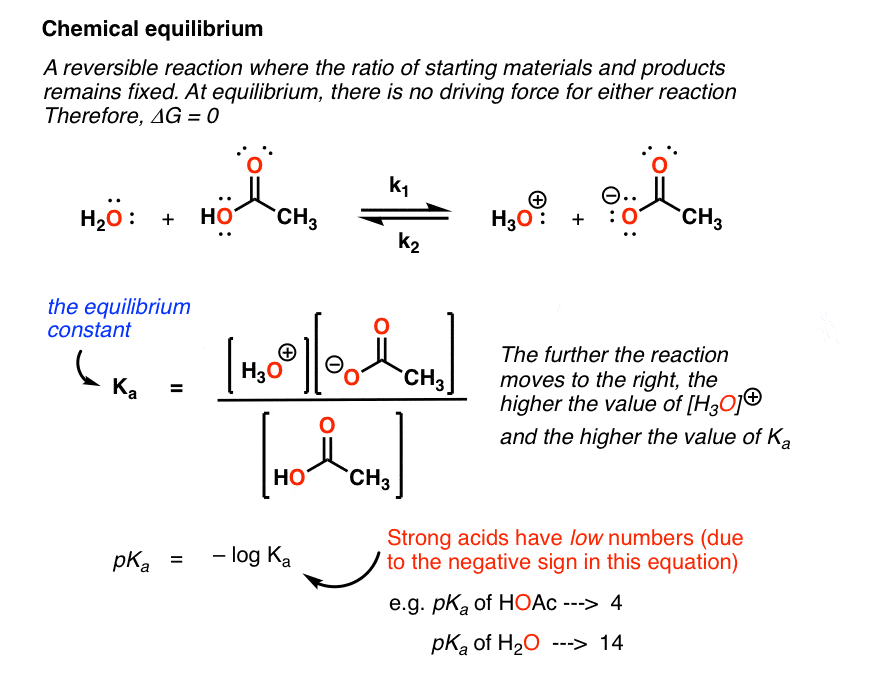It’s important to note that the relative strengths of acids are gauged by the value of this equilibrium constant. This provides us with the extremely useful measure called pKa, which is equal to the negative log of the Ka for this reaction. pKa is a great way to compare the relative acidity of different molecules. As the acidity of molecules increase, this equilibrium will proceed further and further to the right. The result is that strong acids have low pKas (just as strong acids dissolved in water lead to low pH) and weak acids have high pKas. ]

You probably don’t think about it, but chemical reactions in equlibrium are operating around you all the time in such subtle ways you don’t even notice. Inside each of the cans inside the pop machine near you, for instance, equilibria have been established between water and  the (pressurized) carbon dioxide dissolved in solution, which forms carbonic acid; at the same time, molecules of carbonic acid are dissociating to provide carbon dioxide and water.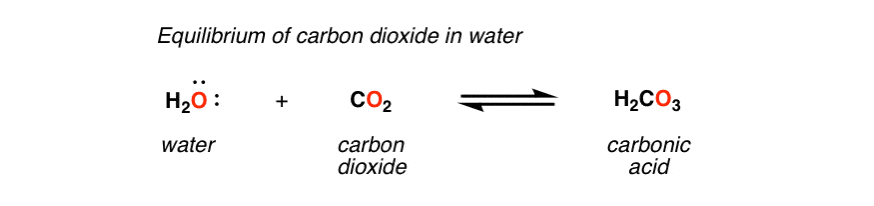Net change in concentration of carbon dioxide, water, and carbonic acid: zero

The equilibrium constant can be used to determine Δ G.

At equilibrium there is no driving force. The Δ G for the reaction is zero.  There is a really useful  numerical relationship between the equilibrium constant and  Δ G, called the Gibbs free energy isotherm equation that we can use to solve for Δ G (if we know the equilibrium constant) or the equilibrium constant (if we know Δ G).

The Gibbs equation:       Δ G = –RT lnK

In your organic chemistry class you’ll see many examples of reversible reactions that lead to  mixtures. [One specific example is in the study of conformations, which are the three dimensional states of molecules formed through the reversible rotation of bonds]. This is how you use the equation to figure out Δ G, if you know K: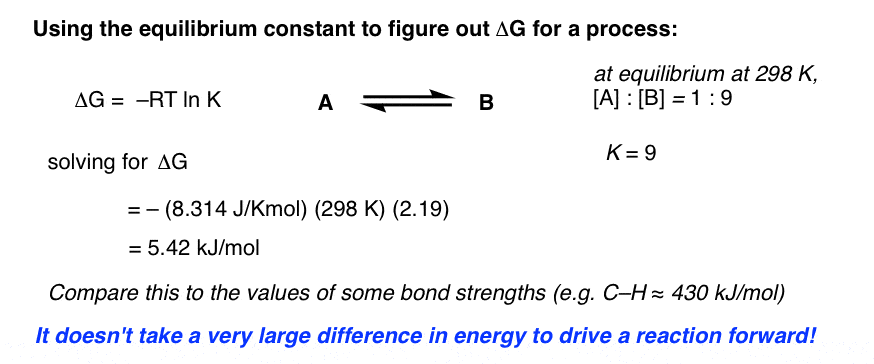You can even plug this equation into graphical form to see the relationship (if you can pardon the crappy Excel skills)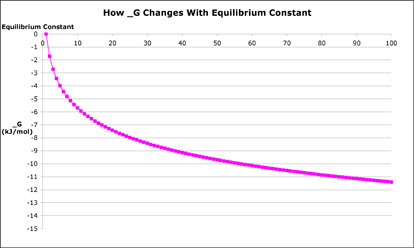Look how steep the beginning part of that curve is! It’s pretty amazing how small the energy difference is for a reaction that gives you a 9:1 mixture of products. When you think about it, 5.4 kJ/mol isn’t very much – compare it to the strength of the C-H bond, for instance, which is 430 kJ/mol. For a reaction to give you a 99:1 ratio, the difference has to be only 11.4 kJ/mol.

The principle of Lé Châtelier

Here’s the final key concept about equilibrium.  Organic chemistry is a practical science. We’re often concerned with transforming one compound into another. We often want to shut down the reverse reaction to improve our yields of product.

We can often find a way manipulate the equilibrium to provide us with products that we desire. You’ll often hear this described as driving the reaction forward. This is done by removing one of the byproducts of the forward reaction, hence preventing the reverse reaction from occurring.

Here’s an example. Treating an aldehyde with an amine generates a compound called an imine as well as a molecule of water. This reaction is reversible; water can react with the imine to give us back the product.

Here’s the key point. If you can find some way to remove the water here, you essentially shut down k2 (the reverse reaction). What’s that going to do to the overall reaction? It will proceed in the forward (rightward) direction until equilibrium is re-established. Which is never, because we’ve added the dessicant. Result: one product, instead of a mixture.

Practically, removal of water can be done in several ways, one of them through the addition of a substance (called a dessicant) that will react irreversibly with  water and therefore remove it from the equation. Magnesium sulfate (MgSO4) is just one example – it forms a hydrate.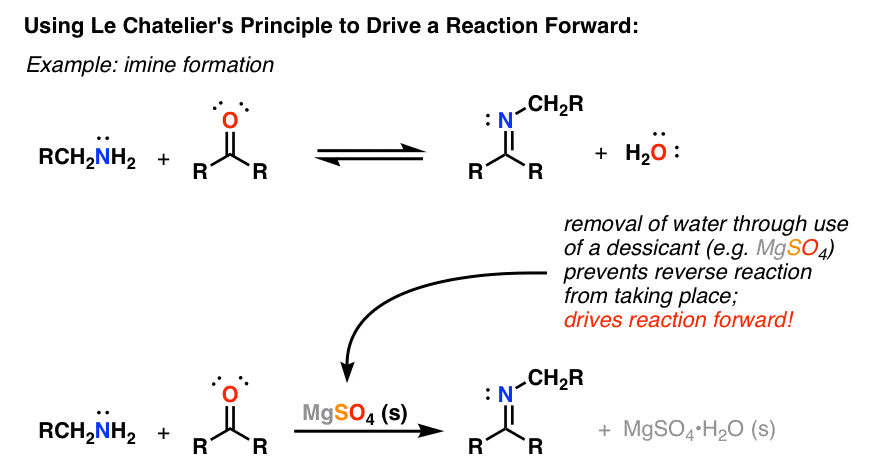The name for this concept should be familiar to you: it’s called Le Chatelier’s principle.

You’ll encounter lots of examples of Le Chatelier’s principle in organic chemistry, since you’ll encounter a lot of reversible reactions (some in Org 1, but a lot in Org 2).

Bottom line: If you understand a reaction and its products, you can choose conditions that allow for the removal of products that will thereby prevent an equilibrium from establishing itself and drive the reaction toward the products you desire.

This is the last lesson in “From Gen Chem to Organic Chem”! I hope you’ll find it a useful resource and guide as you make the transition from the calculation-heavy course that is Gen Chem to the more qualitative realm of organic chemistry. I’ll do a wrapup in the next post.

Next Post – From Gen Chem to Organic Chem, Part 14 – Wrapup!!!!

## Comment section

### 3 thoughts on “From Gen Chem to Organic Chem, Pt. 13 – Equilibria”

1.Katelyn says:

Why do you calculate delta G for an equilibirum reaction if delta G is zero at equilibrium? What is there to calculate?

1.James Ashenhurst says:

That is a *fantastic* question.

The difference is between delta G of the *system* and delta G° (the energy of formation) of the individual components.

For a *system* at equilibrium, delta G is zero. There is no net change of energy.

If the equilibrium constant was 1 (concentration of the products = concentration of reactants) then we could *also* say that the delta G° (delta G of formation) of the products is equivalent to that of the reactants.

But most of the time, the equilibrium constant is greater (or lower) than 1 (depending on which direction you draw the equation). That means one component is more *stable* than the other, and we can relate their relative stabilities to the equilibrium by using the Gibbs free energy equation, delta G° = RT ln K. If we know the equilibrium constant at a given temperature we can rearrange the equation to solve for the difference in energies.

Does that make sense?

2.Alton says:

This clarified that for me. Thank you!

This site uses Akismet to reduce spam. Learn how your comment data is processed.